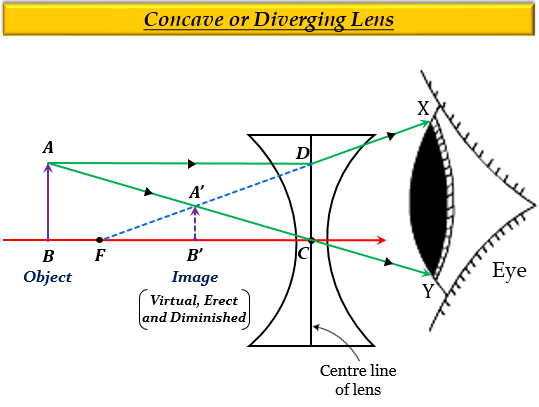# Show by drawing a ray-diagram that the image of an object formed by a concave lens is virtual, erect and diminished.

The image formed by a concave lens is always virtual erect and diminished because it is formed on the left side of the lens.

Let us take an object $AB$ beyond the focus $(F)$ as shown in the figure given below. The image $A'B'$ is formed on the left side and above the principal axis of the concave lens and therefore, it is virtual and erect (upright) in nature. Also, the height of image $A'B'$ is less than the height of object $AB$, hence the image is diminished in size.Updated on: 10-Oct-2022

77 Views